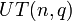# Number of conjugacy classes in unitriangular matrix group of fixed degree over a finite field is polynomial function of field size

## Statement

Suppose$n$ is a natural number. Then, there exists a polynomial function$f$ of degree$n - 1$ such that, for any prime power$q$, the number of conjugacy classes in the unitriangular matrix group$UT(n,q)$ (i.e., the unitriangular matrix group of degree$n$ over the finite field of size$q$) is$f(q)$.

## General observations

Below, we list some general observations about the polynomial in$q$ giving number of conjugacy classes in$UT(n,q)$.

Item Value
Degree of polynomial$n - 1$
Leading coefficient of polynomial positive, value unclear
Factors of polynomial no common factors
Coefficients of polynomial Looks like all coefficients are integers

## Particular cases$n$ (degree of unitriangular matrix group)$n - 1$ (degree of polynomial) polynomial of$q$ giving number of conjugacy classes in$UT(n,q)$ More information
1 0 1 trivial group
2 1$q$ isomorphic to the additive group of the field, hence abelian of order$q$
3 2$q^2 + q - 1$ See element structure of unitriangular matrix group of degree three over a finite field and linear representation theory of unitriangular matrix group of degree three over a finite field
4 3$2q^3 + q^2 - 2q$ See element structure of unitriangular matrix group of degree four over a finite field and linear representation theory of unitriangular matrix group of degree four over a finite field
5 4$5q^4 - 5q^2 + 1$ See element structure of unitriangular matrix group of degree five over a finite field and linear representation theory of unitriangular matrix group of degree five over a finite field
6 5  ?
7 6  ?

There is probably some general formula for these polynomials for all degrees without having to do the entire analysis of element structure and conjugacy classes, but it's unclear what this formula is.